##### Statistical Analysis with Excel For Dummies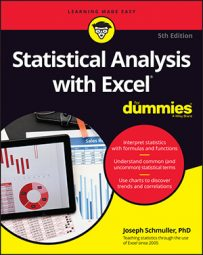Excel offers you functions for working with binomial distribution and negative binomial distribution. These distributions are computation intensive, so let’s get to the worksheet functions right away.

## BINOM.DIST and BINOM.DIST.RANGE

These are Excel's worksheet functions for the binomial distribution. Use `BINOM.DIST` to calculate the probability of getting four 3's in ten tosses of a fair die:
1. Select a cell for `BINOM.DIST`'s answer.
2. From the Statistical Functions menu, select BINOM.DIST to open its Function Arguments dialog box.
3. In the Function Arguments dialog box, type the appropriate values for the arguments. In the Number_s box, enter the number of successes. For this example, the number of successes is 4. In the Trials box, enter the number of trials. The number of trials is 10. In the Probability_s box, enter the probability of a success. Enter 1/6, the probability of a 3 on a toss of a fair die. In the Cumulative box, one possibility is `FALSE` for the probability of exactly the number of successes entered in the Number_s box. The other is `TRUE` for the probability of getting that number of successes or fewer. Enter `FALSE`. With values entered for all the arguments, the answer appears in the dialog box.
4. Click OK to put the answer into the selected cell.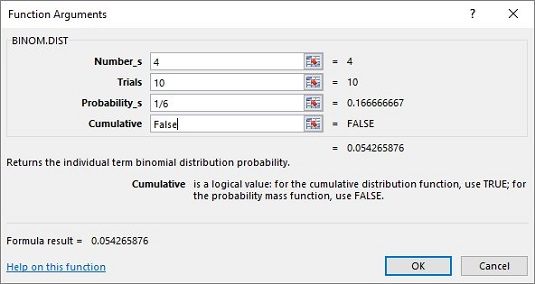To give you a better idea of what the binomial distribution looks like, you can use `BINOM.DIST` (with `FALSE` entered in the Cumulative box) to find pr(0) through pr(10), and then use Excel’s graphics capabilities to graph the results.

Incidentally, if you type TRUE in the Cumulative box, the result is .984 (and some more decimal places), which is pr(0) + pr(1) + pr(2) + pr(3) + pr(4).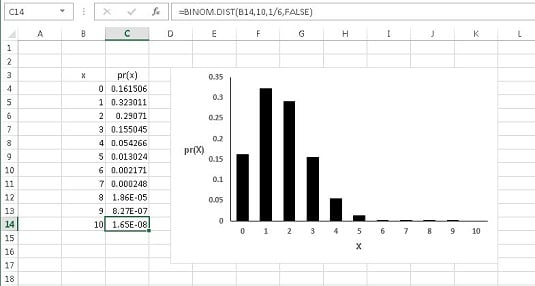The binomial distribution for x successes in ten tosses of a die, with p = 1/6.

The image above is helpful if you want to find the probability of getting between four and six successes in ten trials. Find pr(4), pr(5), and pr(6) and add the probabilities.

A much easier way, especially if you don’t have a chart like the one above handy or if you don’t want to apply `BINOM.DIST` three times, is to use `BINOM.DIST.RANGE`. The image below shows the dialog box for this function, supplied with values for the arguments. After all the arguments are entered, the answer (0.069460321) appears in the dialog box.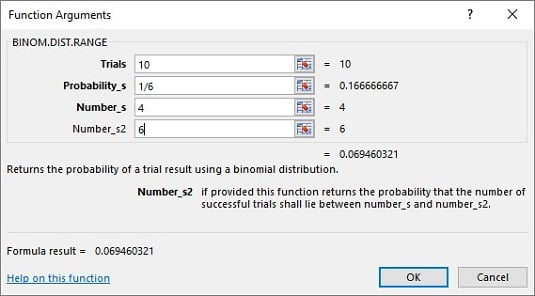The Function Arguments dialog box for `BINOM.DIST.RANGE`.

If you don’t put a value in the Number_s2 box, `BINOM.DIST.RANGE` returns the probability of whatever you entered into the Number_s box. If you don’t put a value in the Number_s box, the function returns the probability of, at most, the number of successes in the Number_s2 box (for example, the cumulative probability).

## NEGBINOM.DIST

As its name suggests, `NEGBINOM.DIST` handles the negative binomial distribution. It’s used here to work out the probability of getting five failures (tosses that result in anything but a 3) before the fourth success (the fourth 3). Here are the steps:
1. Select a cell for `NEGBINOM.DIST`'s answer.
2. From the Statistical Functions menu, select NEGBINOM.DIST to open its Function Arguments dialog box.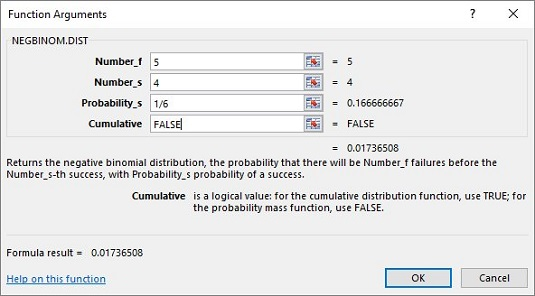3. In the Function Arguments dialog box, type the appropriate values for the arguments. In the Number_f box, enter the number of failures. The number of failures is 5 for this example. In the Number_s box, enter the number of successes. For this example, that’s 4. In the Probability_s box, enter 1/6, the probability of a success. In the Cumulative box, enter `FALSE`. This gives the probability of the number of successes. If you enter `TRUE`, the result is the probability of at most that number of successes. With values entered for all the arguments, the answer appears in the dialog box. The answer is 0.017 and some additional decimal places.
4. Click OK to put the answer into the selected cell.